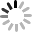# 19级师大 管理会计（本）

1-5   C D D A D   6-10 D D   A   B    C   11-15 A A   B   B D   16-20 B   B   D D   D

1. ABCD   2. ACDE   3. ABD   4. BD   5. AB   6. ABC   7. ABD   8. CD

1.×   2.√   3.√   4.×   5.√   6.×   7.×   8.×   9.√   10.

1、变动成本法：
（1）单位产品成本=10+5+7=22元
（2）期间成本=4000+4×600+1000=7400元
（3）销货成本=22×600=13200元
（4）贡献边际=40×600-（22×600+4×600）=8400元
营业利润=8400-（4000+1000）=3400元

2、完全成本法：
（1）单位产品成本=22+4000/1000=26元
（2）期间成本=4×600+1000=3400元
（3）销货成本=26×600=15600元
（4）营业利润=40×600-15600-3400=5000元

2.1）固定成本=25000/20×6=7500元
7500×12=90000
（2）6/20×100%=30%
[4+7（1+10%）+3]/（1-30%）=21元
（21-20）/20*100%=5%0